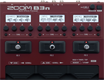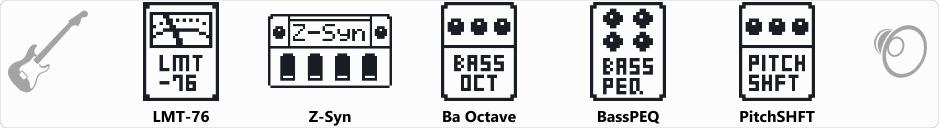# Factory : MJ Synth

Discussion in 'Zoom B3n' started by Tonelib, May 20, 2018.

1. Factory : MJ SynthDevice: Zoom B3n
Firmware: 2.00

Name on device: MJ Synth
Optimized for: Phones/Speaker

Effects chain:This synth bass sound is great for pop.

Effect: "LMT-76" (Dynamics), active - "yes"
"Input" = 40
"Ratio" = 4:1
"REL" = 20
"Output" = 36

Effect: "Z-Syn" (Sfx), active - "yes"
"Frequency" = 5
"Range" = 9
"Decay" = 65
"Resonance" = 11
"Wave" = SAW
"Tone" = 5
"Balance" = 80
"Volume" = 38
"Frequency" = 2
"Range" = 8
"Decay" = 72
"Resonance" = 18
"Wave" = SAW
"Tone" = 7
"Balance" = 80
"Volume" = 80
"Parameter set" = A

Effect: "Ba Octave" (Modulation), active - "yes"
"Oct" = 16
"Lo" = 2
"Hi" = 5
"Dry" = 95

Effect: "BassPEQ" (Filter), active - "yes"
"Frequency" = 200Hz
"Q" = 1.0
"Gain" = 2.5
"Volume" = 80

Effect: "PitchSHFT" (Modulation), active - "yes"
"Shift" = 12
"Fine" = 0
"Tone" = 4
"Balance" = 2

Patch Volume: 70